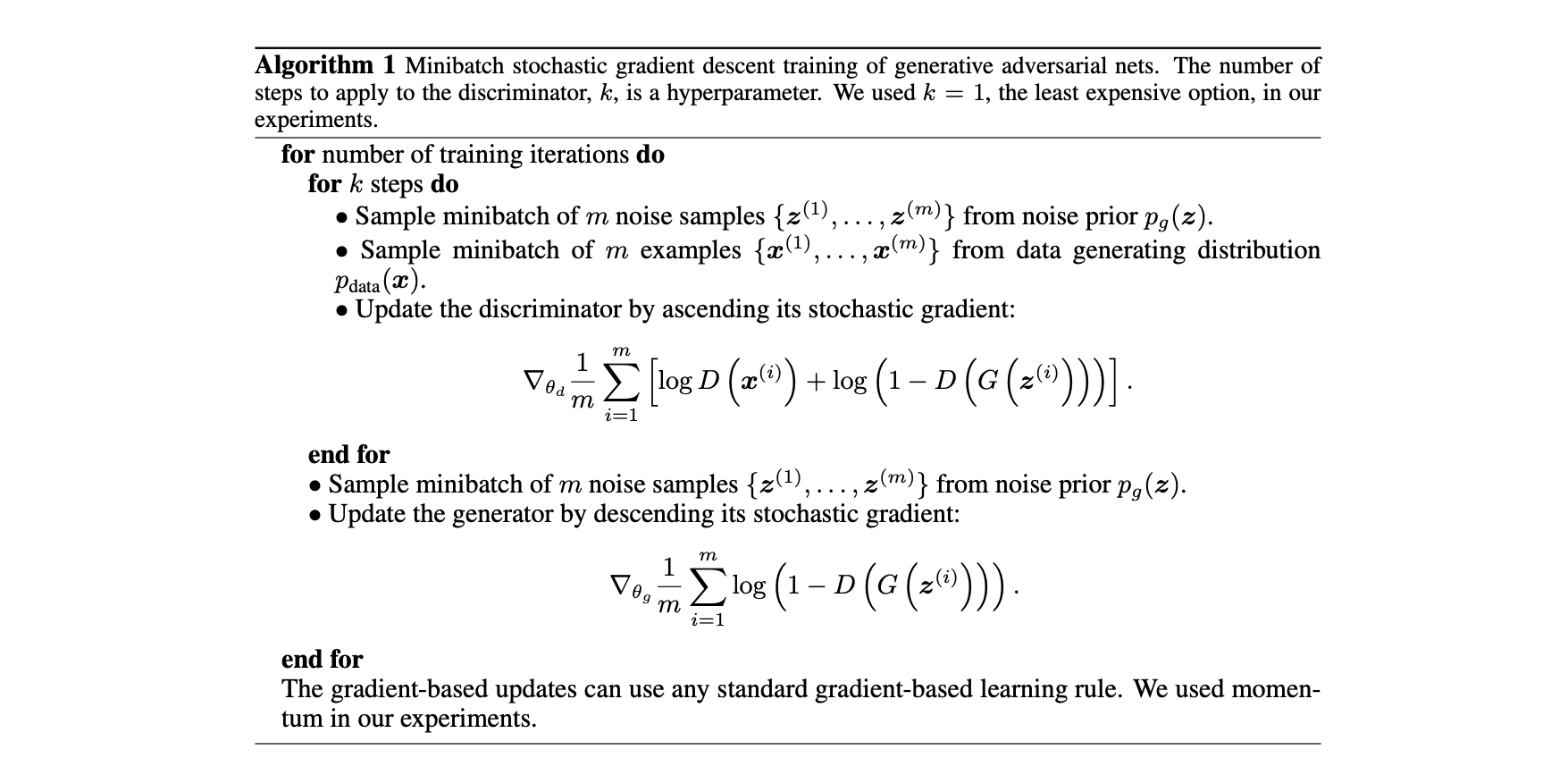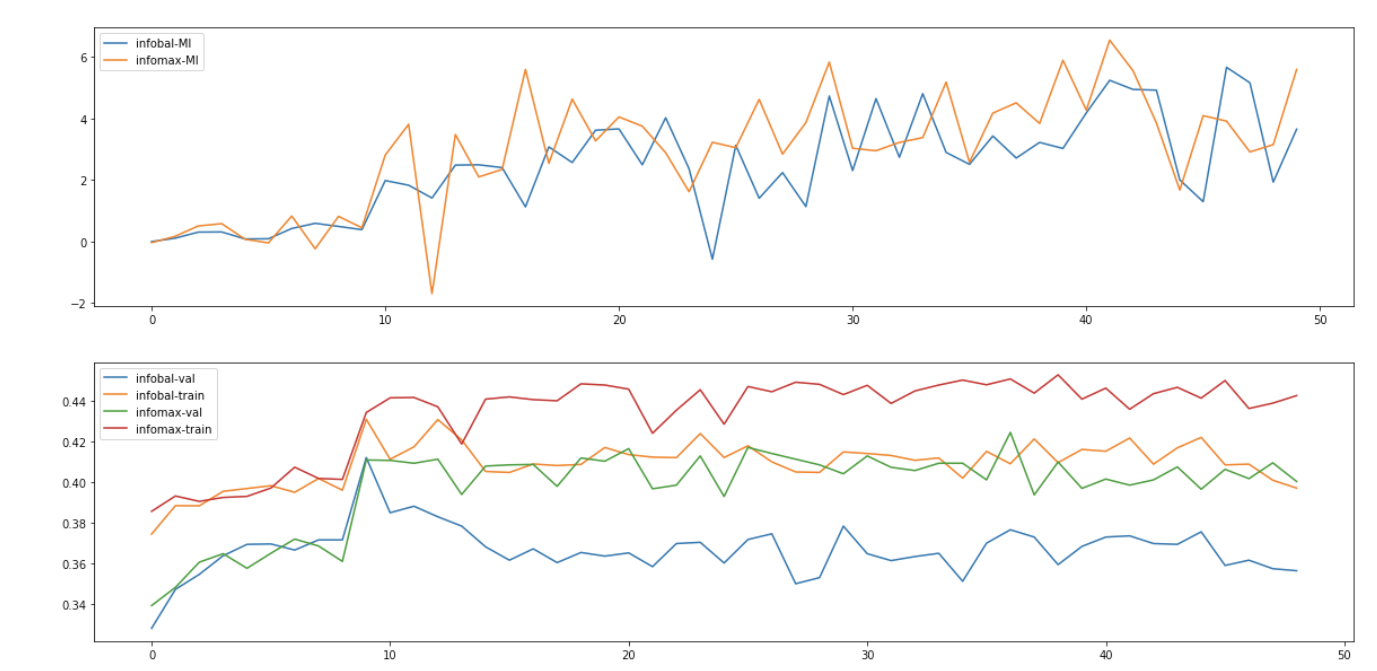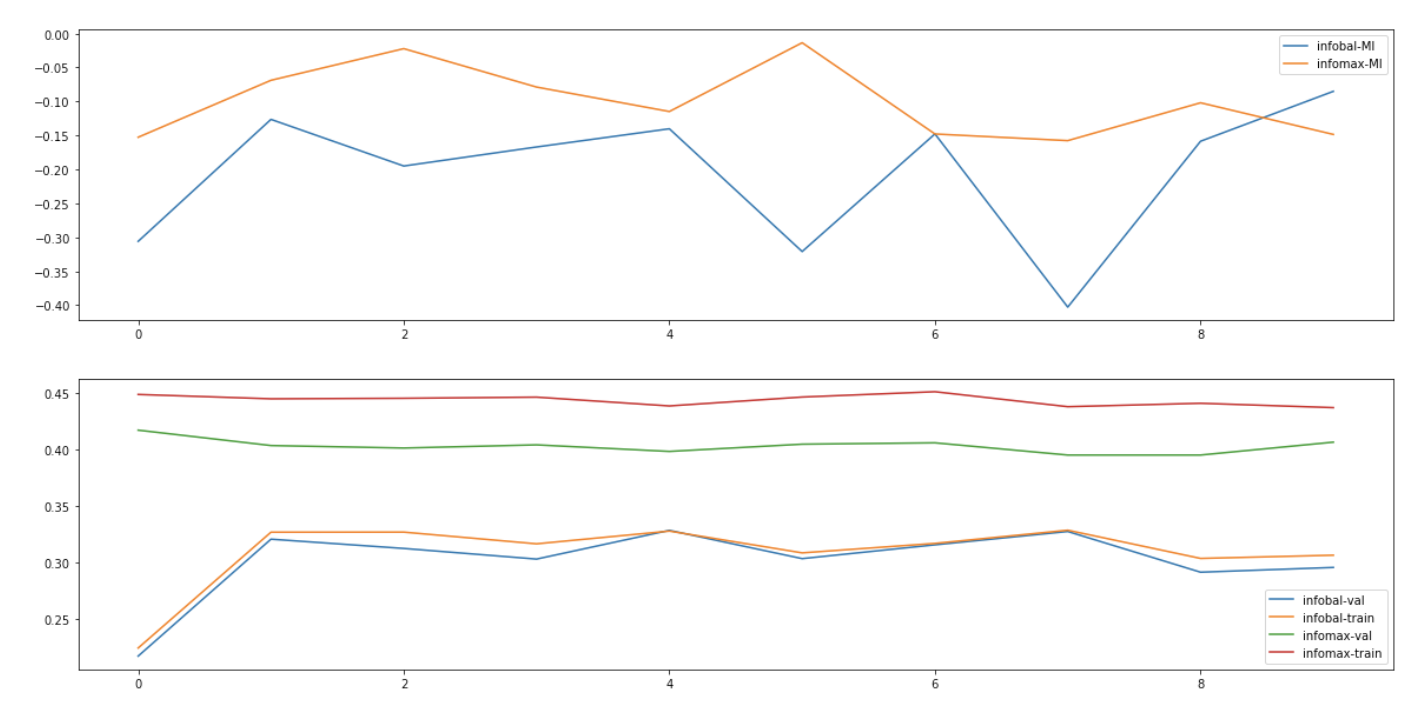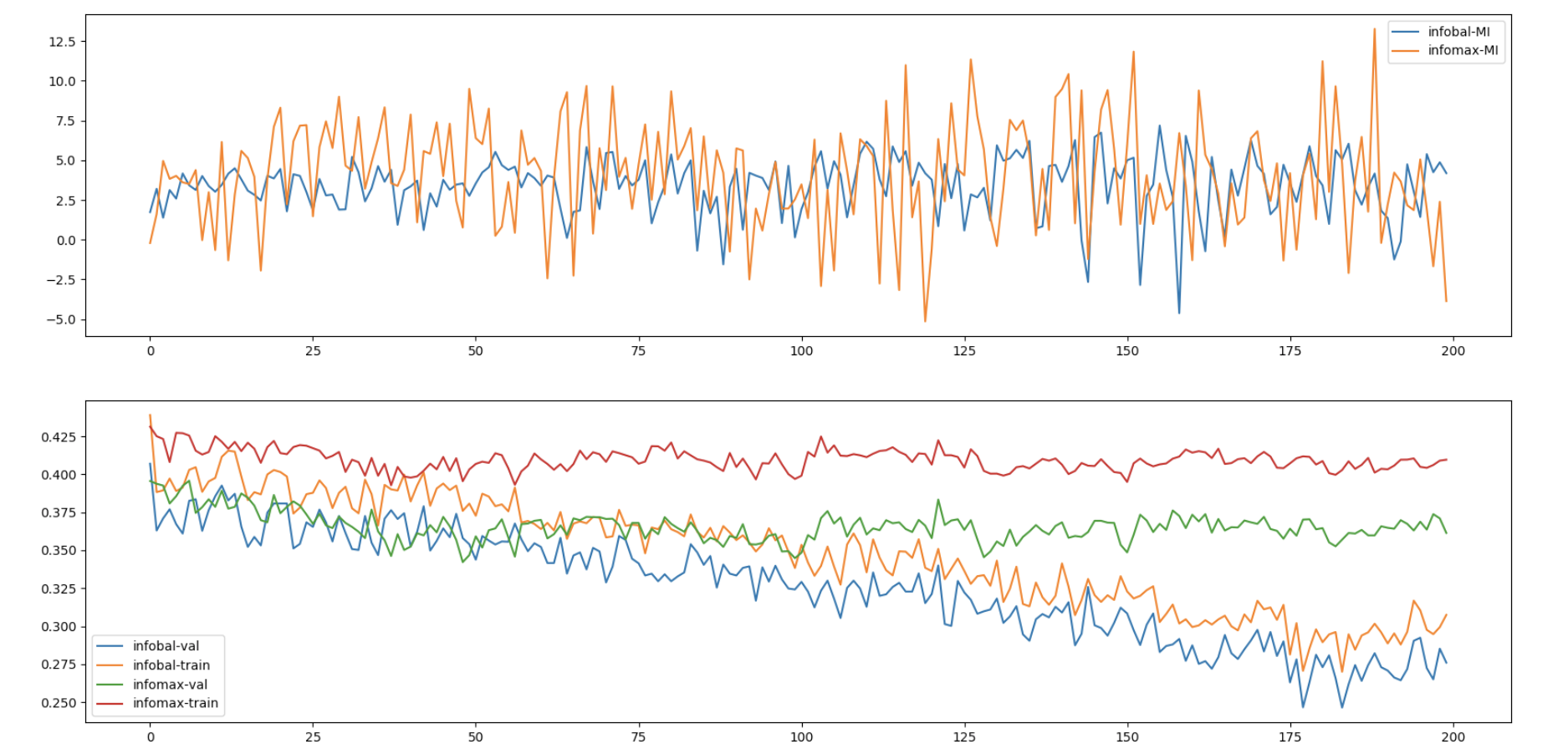N1H111SM's Miniverse

# InfoBal - Ideas (failed)

2020/05/26 ShareMaterials

# Motivation

CMC的动机在于：重要的信息在多个view中被共享，所以我们应当最大化多个view的mutual information. 在CMC作者的延续性工作中，他认为只考虑view-invariant的信息是不够的，最佳的信息应当是和下游任务高度相关的，因此提出了InfoMin principle, 其核心思想是在input的两个view连同整体input和下游任务标签的MI保持一致的前提下，尽量使两个view之间的MI降低。而ICLR-2020的文章则将以往提出的三个MI优化目标（分别为global/local MI, CMC, CPC）都归入了统一的multiview理论框架下，认为这三个优化目标是这个框架下平行的优化目标。

# Theory

## Problem Description

Suppose the input dataset is $X$. Consider the simplest setting of only 2 views $V_1$ and $V_2$ of $X$, which is parameterized by $\theta_1$ and $\theta_2$:

In original InfoMax principle, the objective of learning representation of single view is to maximize:

When there are multiple views involved, we want them to be simultaneously maximized:

In InfoMin principle, the objective is to minimize the MI between different views under certain (complicated) constraints.

The above two objectives without constraints may not be so favorable analytically, since they both have trivial solutions which result in useless representation.

• For the original InfoMax principle: let function $g$ be bijective function, all the information of $X$ is preserved and the MI is maximized, no good form of representation is learned.
• For the multiview InfoMin principle: let $V_1$ and $V_2$ output constant vectors, and their MI is 0, which is minimized.

## InfoBal Objective

To solve this problem, notice that there exists an inequality:

which yields:

So we can change our objective of representation learning with $M$ views to maximizing the following:

where $\Theta=\{\theta_1, \theta_2, \cdots, \theta_M\}$ represents the set of the view encoders, and is guaranteed bounded.

The reason why this formula is better than previous ones is that it helps the model to balance the information capacity and multiview diversity of the representation. And also in the two extreme cases where two single objectives can’t deal with morbid representation, the new objective function equally hates them:

In the first morbid scenario, when every $V_i$ preserves all the info about $X$, i.e.,

which is quite favorable, since the views (representations) are super powerful. While without constraints, we can assume the happening of the worst: each view is exactly identical, which makes the multiview setting not learning diverse representations. This case is discouraged by our new objective:

In the second morbid scenario, when MI across the views are minimized, which means $V_i$ is irrelevant to each other, i.e., $I(V_i, V_j)=0$, this InfoMin situation could lead to extreme loss of information:

while in our objective, this is also discouraged:

## Global/Local InfoBal

The final representation $R$ is the result of view aggregation:

In global/local MI maximization, the target is to maximize the Mutual Information between the global and the local representation of the data:

which lacks theoretical derivation why this is a more favorable objective. We could also do this adaptation to our new objective by replacing original input $X$ with final representation $R$:## Weighted InfoBal

Another potential optimiztion for InfoBal is to assign two weight matrix: view weight and correlation weight (这个名字不确定).

## Optimizing InfoBal

One way is to adversarially optimize the InfoBal training objective, since the InfoMin is a minmax problem.Another way is to found the upper bound of the objective and minimize the upper bound, which is introduced in another similar paper (refer to NIPs-2019 paper “Information Competing Process for Learning Diversified Representations”). This one involves the knowledge of variational inference, which will be investigated in the future.

# Experiments

• View Encoder: 从raw input进行多个view的编码模块
• View Aggregator: 从view representation聚合成最终representation的模块
• InfoMax critic: 用于MI maximization的parameterized DV representation模块
• InfoMin discriminator: 最小化view之间的MI的discriminator模块We conducted the experiments on CIFAR10 dataset using the 2-view setting: instead of simultaneously maximizing the MI between the representation and the feature map vectors, we split the feature map into top/bottom parts and maximize the MI.

To evaluate the quality of the final representation, we plug the representation into a one-layer neural classifier as DIM does. We train the classifier for 4 epochs, which is by test converged, and evaluate the accuracy of the classifier.Change into JSD critic function, and we have the result:When trained in much longer period, we can even find out that the InfoBal objective is constantly doing harm to the representation quality.The figure above is the experimental result, about which we have some questions and concerns:

• The lower bound of MI is increasing along training, while the downstream task accuracy is not improved (as much) as the MI estimate.
• The improvement compared to the original starting points is rather marginal.
• InfoBal objective is constantly hurting the performance of the representation learning model, despite the fact that the lower bound MI estimate value is nearly the same, which also implies that the MI is not so relevant to the representation quality.

# Conclusion

Up to now, we can conclude that the new objectice InfoBal is a failed attempt. One thing worth trying though, is to reimplement the DIM’s global/local objective in multiple (way more than 2) views and shed some light on our future exploration.